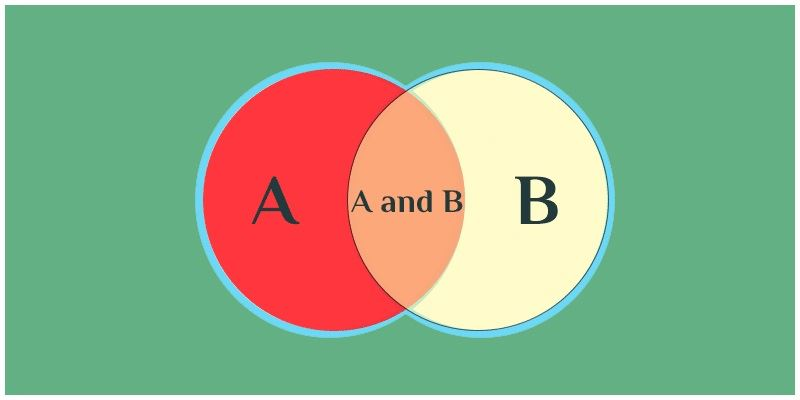Events In Probability

Algebra of Events

Number of favorable outcomes to the total number of outcomes is defined as the probability of occurrence of any event.Certain operations we perform on sets are applicable to probability. Two or more events can be combined using various operations as union, intersection, complement and so on. Let us study about these operations in the upcoming discussion.Let us take events associated with any random experiment having the sample space S.

i) Complementary Event: For any event E1 there exists another event E1 which represents the remaining elements of the sample space S.

If a dice is rolled then the sample space S is given as S = {1 , 2 , 3 , 4 , 5 , 6 }. If event E1 represents all the outcomes which is greater than 4 then E1 = {5,6} then, E1 = {1,2,3,4}.

Thus E_1 is the complement of the event E1. Similarly the complement of E1, E2, E3……….En

will be represented as E1, E2, E3………En.

ii) Events associated with “OR”: If two events E1 and E2 are associated with OR then it means that either E1 or E2 or both. The union symbol(∪) is used to represent OR in probability.

Thus the event E1, E2 denotes E1, E2.

If we have mutually exhaustive events E1,E2,E3………En associated with sample space S then,

E1, E2, E3………En = S

iii) Events associated with “AND”:If two events E1 and E2 are associated with AND then it means the intersection of elements which is common to both the events. The intersection symbol(∩) is used to represent AND in probability.

Thus the event E1, E2 denotes E1 and E2.

iv) Event E1 but not E2: It represents the difference of both the events. E1, E2 represents all the outcomes which are present in E1 but not in E2. Thus the event E1 but not E2 is represented as

E1, E2 = E1 E2

To have a better insight let us consider an example:

Example: In the game of snakes and ladders a fair die is thrown. If event E1 represents all the events of getting a natural number less than 4, event E2 consists of all the events of getting an even number and E3 denotes all the events of getting an odd number. List the sets representing the following:

i)E1 or E2 or E3

ii)E1 and E2 and E3

iii)E1 but not E3

Solution:

The sample space is given as S = {1 , 2 , 3 , 4 , 5 , 6}

E1 = {1,2,3}

E2 = {2,4,6}

E3 = {1,3,5}

i)E1 or E2 or E3= E1 E2 E3= {1, 2, 3, 4, 5, 6}

ii)E1 and E2 and E3 = E1 E2 E3 = ∅

iii)E1 but E3 = E1 E2= {2}

This is all about algebra of events associated with probability. To learn more about probability problems and probability formulas, please visit our website www.byjus.com. We wish you a happy learning!!!